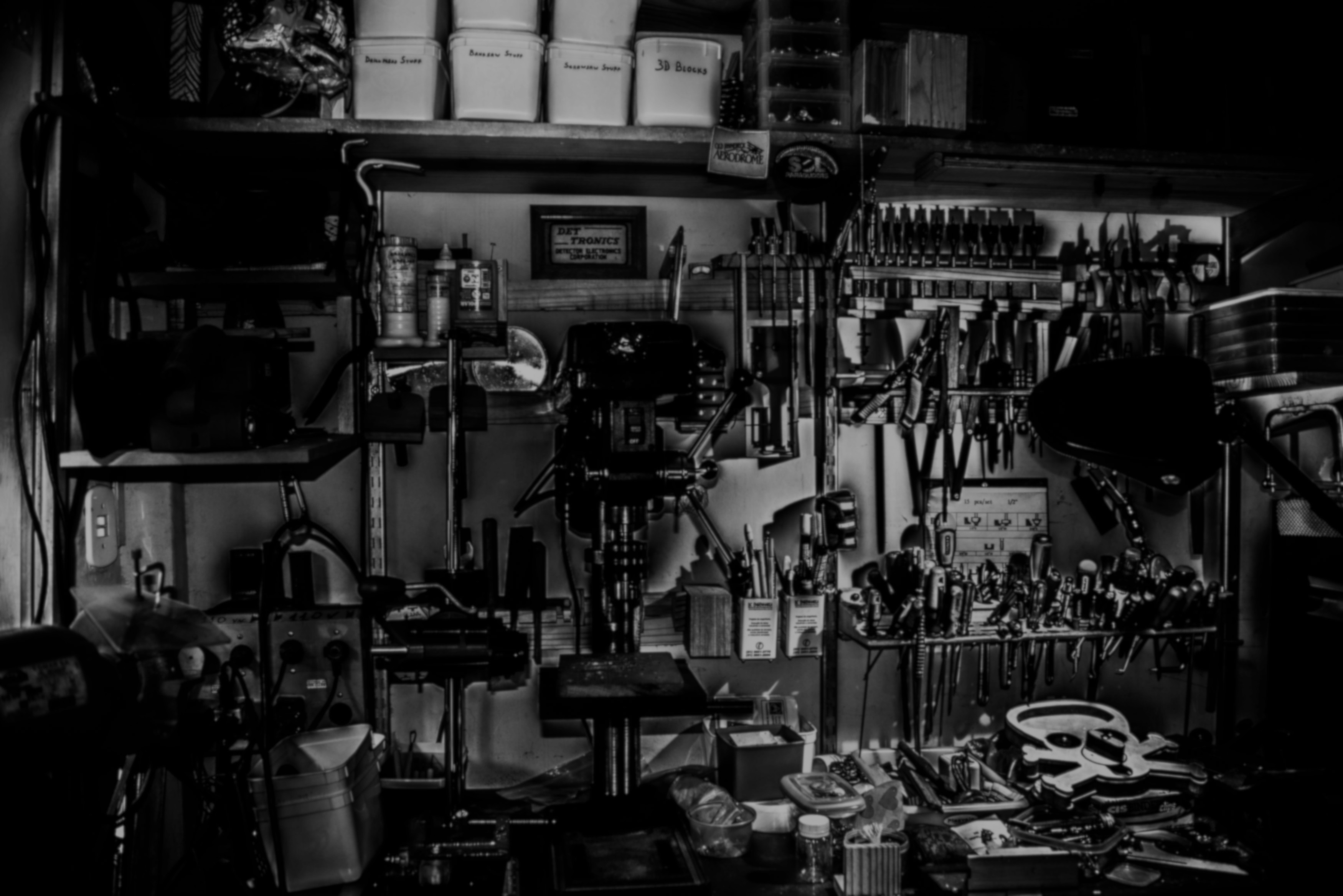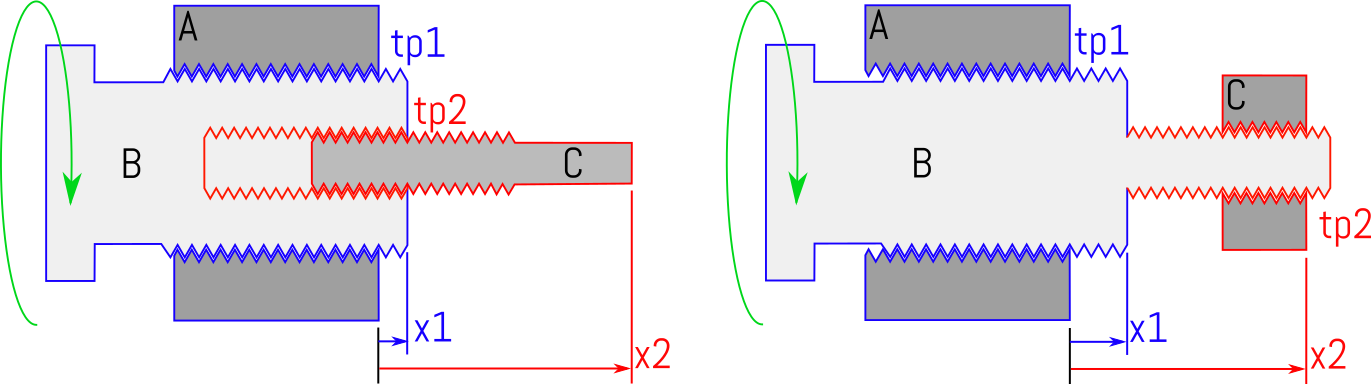# Mechanomy# Monthly Mechanism: Differential Screws

What happens if you put a screw inside a screw?

Today we're kicking off a regular series on interesting mechanisms, starting with the differential screw; a very compact mechanism for generating very fine motions. Their small size makes them a bit hard to spot in-the-wild, but these are commonly seen in precise positioners, able to scale finger-knob rotations down to nanometer displacements. For instance, ThorLab's nanoMax stages uses 3 differential screws to drive a kinematic stage with nanometer precision.

Differential screws are mechanically simple and very compact for their mechanical advantage, consisting of three parts related through two threads. They are usually seen in two primary configurations:Parts A, B, and C interface through thread pitches tp1 and tp2 to form a differential screw.

In the left configuration, the internally threaded part A is assumed fixed, dual-threaded part B is rotated by some user, while externally-threaded part C is free to translate while prevented from rotation. The right configuration merely gives C the internal thread and B two external threads; other permutations are possible but often more challenging to produce. Parts A and B interface through thread pitch 1 (tp1), and B and C through thread pitch 2 (tp2).

## Calculating travel #

Converting threads per inch into length per radian, $p = 1 /(2\pi tpi)$, rotating B causes it to translate with

$x_1 = \theta / tp_1 = \theta p_1$.

As C can only translate, B's rotation also causes a displacement between B and C of

$x_2 - x_1 = \theta / tp_2 = \theta p_2$.

Rearranging we find

$x_2 = \theta (p_1 + p_2) = \theta p_{eff}$.

This is rather hum-drum; where it gets more interesting is in the choice of the thread pitches. The 'differential' screw refers to the common case where tp1 and tp2 differ in their handedness, say tp1 = 24 threads per inch right-handed, and tp2 = 28 threads per inch left-handed. Representing right handed threading as positive and left as negative, converting units

$p_1 = 1/(2*\pi*24) = 1/150.7 in/rad$

and

$p_2 = 1/(2*\pi*-28) = -1/175.9 in/rad$.

Giving $x_2 = \theta (1/150.7 - 1/175.9 ) = \theta * 9.51e-4 in/rad$, which for a full turn $x_2 = 5.598e-3 in = 0.151mm$, all with two common threads.

Physically, we would see that turning B to the right (positive) causes it to translate to the right, while the left-handed threads on C would cause it to screw into B. If instead we had made tp2 right-handed and tp1 left, this would have produced a negative effective pitch, causing B to translate left and C to emerge from B. As it is only the thread difference that matters, it is trivial to make a table of common thread pitches and resulting, effective pitch: